## Characteristics of Negative Feedback Amplifiers:

The Characteristics of Negative Feedback Amplifiers are given below

### Stabilization of Gain With Negative Feedback:

The variations in temperature, supply voltages, ageing of components or variations in transistor parameters with replacement are some of the factors that affect the gain of an amplifier and cause it to change. However, the overall gain of the amplifier can be made independent of these variations if negative feedback is used. This is an important advantage of negative feedback.

From Eq. (19.8), the voltage gain with negative feedback is given as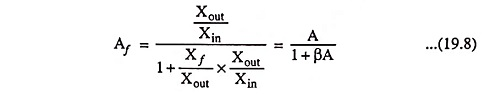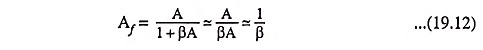if Aβ is made much larger than unity

The gain is thus independent of internal gain of the amplifier and depends on the passive elements such as resistors. The values of resistors remain fairly constant because they can be chosen very precisely with almost zero temperature coefficient of resistance. Thus the gain is stabilized.

Even if open-loop gain A is not very large, some improvement in gain stability can be achieved.

Differentiating Eq. (19.8) w.r.t. A, we have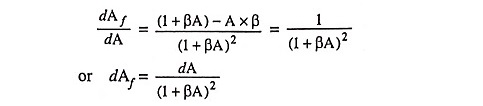Dividing above equation by Eq. (19.9), we have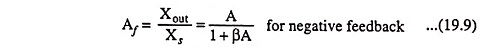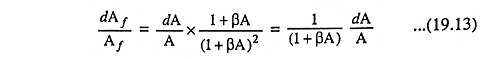The term dAf/Af represents the fractional change in amplifier transfer gain with feedback and  dA/A denotes the fractional change in transfer gain without feedback. The term 1/1 + Aβ called the sensitivity of the transfer gain.

Thus, the sensitivity of the transfer gain is defined as the ratio of percentage change in transfer gain with feedback to the percentage change in transfer gain without feedback.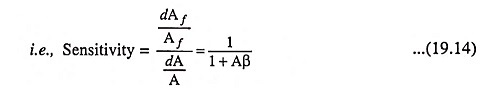The reciprocal of the term sensitivity is called the desensitivity, which is given as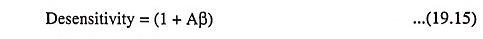In negative feedback (1 + βA) >> 1, the percentage change in gain with negative feedback is less than the percentage change in gain without feedback. Thus negative feedback improves the gain stability of the amplifier.

 Table 19.2    Voltage and Current Signals in Feedback Amplifiers Type of Feedback Signal or Ratio Xout Xs, Xf, Xd A β Voltage-Series Feedback Voltage Voltage Av Vf/Vout Voltage-Shunt Feedback Voltage Current Rm If/Vout Current-Series Feedback Current Voltage Gm Vf/Iout Current-Shunt Feedback Current Current Ai If/Iout

The topology determines which transfer ratio (Table 19.2) is stabilized. For instance, for voltage-series feedback Eq. (19.12) signifies that Afv ≈ 1/β and it is the voltage gain which is stabilized. Similarly, in cases of voltage-shunt feedback, current-series feedback and current-shunt feedback transresistance gain Rm, transconductance gain Gm and current gain Ai respectively are stabilized.

### Reduction in Frequency Distortion With Negative Feedback:

For a negative feedback amplifier having βA >> 1 the gain with feedback is given as Af ≡ 1/β. It follows from this that if the feedback network is pure resistive, the gain with feedback becomes independent of frequency even though the basic amplifier is frequency dependent. Practically, the frequency distortion arising because of varying amplifier gain with frequency is considerably reduced with negative feedback.

### Reduction in Nonlinear Distortion With Negative Feedback:

In this characteristics of negative feedback amplifiers, Harmonic distortion occurs when a transistor or other device is driven beyond its range of linear operation. Now negative feedback is used and the strength of the input signal is increased by the same factor by which the gain is reduced. Thus the magnitude of output signal remains unaltered and thus there is a reduction in harmonic or nonlinear distortion. It may be proved as below.

Let the amplifier with open-loop gain A produce a distortion D in the output signal without feedback. Now when feedback is applied, output as well distortion is fed back to the input. Let the gain with feedback be Af and distortion in the output Df. A part βDf of this distortion is fed back to the input. It gets amplified by factor A and becomes βADf.

Net distortion,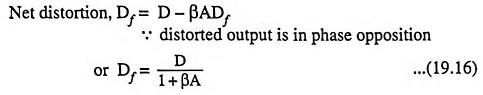Thus the distortion is reduced by the same factor as the gain.

However, it is to be noted that improvement in distortion is possible only when the distortion is introduced by the amplifier itself, not when it is already present in the input signal.

### Reduction in Noise With Negative Feedback:

In this characteristics of negative feedback amplifiers, Noise in an amplifier is reduced in the same way as distortion. However, distortion is usually introduced in the last stage of the amplifier and, therefore, is always within the feedback loop whereas noise in an amplifier may be generated or introduced from outside source at any stage that may not be within the feedback loop. The noise can be reduced by negative feedback only if its source lies within the feedback loop after the first stage.

As nonlinear distortion generated within the amplifier is reduced with negative feedback by a factor (1 + βA), the noise introduced within amplifier also gets reduced by the same factor (1 + βA) on using negative feedback and the performance of the amplifier is thus much improved.

Scroll to Top